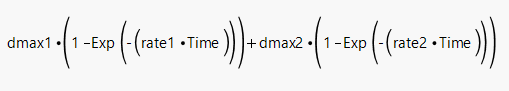Choose Language Hide Translation Bar
Highlighted

## Parameter Constraints in Nonlinear Model Fitting

I am fitting a bi-exponential growth model of the following form:I would like to constrain the parameter estimates so that dmax1< dmax2 and rate1<rate2.

Is there any way to do this in the Nonlinear platform?

6 REPLIES 6
Highlighted

## Re: Parameter Constraints in Nonlinear Model Fitting

If you believe that assumption is true, then the best model should find such estimates without constraint, I think. The data are evidence against that assumption otherwise.

the custom model would remain the same. I think that you would have to introduce the constraint as a penalty to the loss function. The penalty is high when the constraint is violated.

Learn it once, use it forever!
Highlighted

## Re: Parameter Constraints in Nonlinear Model Fitting

This is more a matter of convenience that mathematics.  The actual problem is that I am fitting a non-linear model to several kinetic profiles generated as part of a DOE.  The non-linear model I showed is the theoretical model that we believe describes the process, which consists to two separate reactions, one that is "slow" and another that is "fast".  The slow reaction rate is approx 1/100 of the fast reaction rate.

The problem is that when I fit the non-linear model to each profile, sometimes the "rate1" parameter that estimated is the smallest, and other times the "rate1" parameter is the largest. JMP doesn't know that I want rate1 to be the slow rate.  When I save the parameter estimates, I have to do some data processing to determine which rate is the slow rate and which rate is the fast rate.  Ultimately I want to use the factors in the DOE to predict the slow reaction rate and the fast reaction rate.

Part of me thinks, though, that forcing this constraint may also make the model fits more stable?  But maybe not.  I'm not sure.

Highlighted

## Re: Parameter Constraints in Nonlinear Model Fitting

Sue Walsh talked about this type of model in a paper at JMP Discovery a while back.

The notes in the slides about segmented models are a little sparse, but I think you'll be able to get the gist of how to set it up.

JMP Systems Engineer, Pharm and BioPharm Sciences
Highlighted

## Re: Parameter Constraints in Nonlinear Model Fitting

Thanks for that reference @Byron_JMP .  In this case, it's not a segmented model.  The actual physical process is two reactions happening simultaneously at two different rates, producing the same output result.  The measured output is the sum of the two reaction outputs, but each reaction happens in the same reaction system, so you can't measure them indepedently.

Highlighted

## Re: Parameter Constraints in Nonlinear Model Fitting

I wonder if it would be easier to process the estimates after the nonlinear fitting without contstraints. I assume that the dmax1 and rate1 parameter estimates will go together and the dmax2 and rate2 parameter estimates will go together even if the dmax1 and rate1 parameter estimates are not always first. Then to achieve the order that you want, you can simply swap the order in the data table after saving the estimates table. This example shows what I have in mind:

``````Names Default to Here( 1 );

dt = New Table( "Double Exponential Models",
New Column( "dmax1",
Values( J( 20, 1, Random Uniform() ) )
),
New Column( "rate1",
Values( J( 20, 1, Random Uniform() ) )
),
New Column( "dmax2",
Values( J( 20, 1, Random Uniform() ) )
),
New Column( "rate2",
Values( J( 20, 1, Random Uniform() ) )
)
);

Wait( 5 );

For Each Row(
If( :rate1 > :rate2,
temp = :dmax1;
:dmax1 = :dmax2;
:dmax2 = temp;
temp = :rate1;
:rate1 = :rate2;
:rate2 = temp;
);
);``````
Learn it once, use it forever!
Highlighted

## Re: Parameter Constraints in Nonlinear Model Fitting

I had wrote some JSL that was nearly identical to that, and it did solve the problem.  Thanks for sharing.

Article Labels

There are no labels assigned to this post.